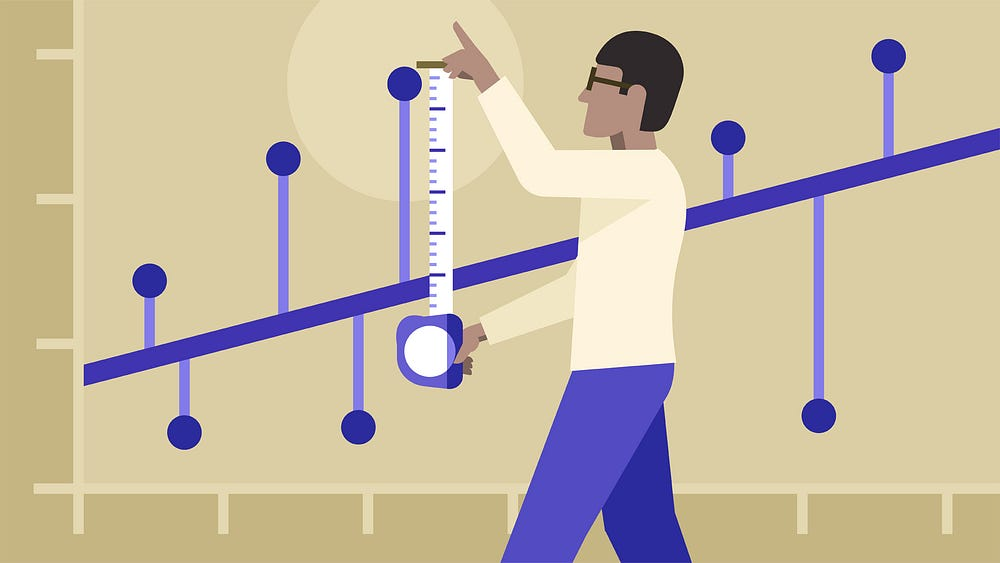# Chapter 2 — Introduction to Linear Regression

Original article was published on Deep Learning on Medium# Regression Analysis

It statistical tool used in Supervised Machine Learning. It is used to find out the most accurate line describing the relationship between a dependent variable(y) and an independent variable(x) with least error. It is used when dependent variable(y) is continuous (2.3,350,50) while, independent variable could be of any type — continuous , nominal or categorical.

# Linear Regression — What is it ? When to use ? When not to use ?

## What is Linear Regression?

Linear Regression takes a linear approach to modelling the relation between dependent and one or more independent variable.

If we consider a single independent variable (x) for predicting a quantitative response (having discrete values) of dependent variable (y). Linear regression assumes approximately a linear relation between x and y as shown below:-

` y= mx + c where: m =slope or gradient c= intercept`

In higher dimensions when we have more than one predictor variable the line is called hyper-plane. Equation becomes :-

` y= β0+ β1x1 + β2x2 +.....+βpxp + εwhere: β0 = intercept p= number of predictions ε =Error`

## When to use Linear Regression ?

1. It can only used when the response variable is continuous.
2. Dependent variable (y) has linear relation with independent variable (x). To check if this condition is satisfied observe the following steps:-

* X-Y scatter plot (graph used to display the relation between two quantitative variables) is linear.

* Residual plot (residual is the difference between observed response (given) and response value predicted by our linear model) shows a random pattern.

3. For each value of x the probability distribution of y has the standard deviation σ . When this condition is satisfied, the variability of the residual will be relatively constant across all values of x, this can be verified using residual plot.

## When not to use Linear Regression ?

1. It cannot be used for more than two categories or levels that is, when we have categories like low risk , medium risk or high risk in our independent variables .
2. Impact of outliers(values that deviates significantly from other values), for large or small value of outliers we need to change the gradient to accommodate the value. This might lead to wrong predictions.

To summarize the blog, we can now explain different types of machine learning algorithms, define regression analysis and got a brief understanding of what linear regression is, when to use and when not to use the method.